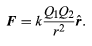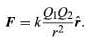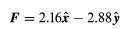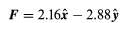Electricity & Electrostatics

# Electricity & Electrostatics - Notes | Study Basic Physics for IIT JAM - Physics

 1 Crore+ students have signed up on EduRev. Have you?

Electricity:

Electricity, phenomenon associated with stationary or moving electric charges. Electric charge is a fundamental property of matter and is borne by elementary particles. In electricity the particle involved is the electron, which carries a charge designated, by convention, as negative. Thus, the various manifestations of electricity are the result of the accumulation or motion of numbers of electrons.

Electrostatics:

Electrostatics is the study of electromagnetic phenomena that occur when there are no moving charges—i.e., after a static equilibrium has been established. Charges reach their equilibrium positions rapidly because the electric force is extremely strong. The mathematical methods of electrostatics make it possible to calculate the distributions of the electric field and of the electric potential from a known configuration of charges, conductors, and insulators. Conversely, given a set of conductors with known potentials, it is possible to calculate electric fields in regions between the conductors and to determine the charge distribution on the surface of the conductors. The electric energy of a set of charges at rest can be viewed from the standpoint of the work required to assemble the charges; alternatively, the energy also can be considered to reside in the electric field produced by this assembly of charges. Finally, energy can be stored in a capacitor; the energy required to charge such a device is stored in it as electrostatic energy of the electric field.

Coulomb’s law:

Static electricity is a familiar electric phenomenon in which charged particles are transferred from one body to another. For example, if two objects are rubbed together, especially if the objects are insulators and the surrounding air is dry, the objects acquire equal and opposite charges and an attractive force develops between them. The object that loses electrons becomes positively charged, and the other becomes negatively charged. The force is simply the attraction between charges of opposite sign. The properties of this force were described above; they are incorporated in the mathematical relationship known as Coulomb’s law. The electric force on a charge Q1 under these conditions, due to a charge Q2 at a distance r, is given by Coulomb’s law,(1)
The bold characters in the equation indicate the vector nature of the force, and the unit vector is a vector that has a size of one and that points from charge Q2 to charge Q1. The proportionality constant k equals 10−7c2, where c is the speed of light in a vacuum; k has the numerical value of 8.99 × 109 newtons-square metre per coulomb squared (Nm2/C2). Figure 1 shows the force on Q1 due to Q2. A numerical example will help to illustrate this force. Both Q1 and Q2 are chosen arbitrarily to be positive charges, each with a magnitude of 10−6 coulomb. The charge Q1 is located at coordinates x, y, z with values of 0.03, 0, 0, respectively, while Q2 has coordinates 0, 0.04, 0. All coordinates are given in metres. Thus, the distance between Q1 and Q2 is 0.05 metre.
The magnitude of the force F on charge Q1 as calculated using equation (1) is 3.6 newtons; its direction is shown in Figure 1. The force on Q2 due to Q1 is −F, which also has a magnitude of 3.6 newtons; its direction, however, is opposite to that of F. The force F can be expressed in terms of its components along the x and y axes, since the force vector lies in the xy plane. This is done with elementary trigonometry from the geometry of Figure 1, and the results are shown in Figure 2. Thus,(1)(2)

in newtons. Coulomb’s law describes mathematically the properties of the electric force between charges at rest. If the charges have opposite signs, the force would be attractive; the attraction would be indicated in equation (1) by the negative coefficient of the unit vector r̂. Thus, the electric force on Q1 would have a direction opposite to the unit vector and would point from Q1 to Q2. In Cartesian coordinates, this would result in a change of the signs of both the x and y components of the force in equation (2).(2)

How can this electric force on Q1 be understood? Fundamentally, the force is due to the presence of an electric field at the position of Q1. The field is caused by the second charge Q2 and has a magnitude proportional to the size of Q2. In interacting with this field, the first charge some distance away is either attracted to or repelled from the second charge, depending on the sign of the first charge.

Superposition Principle:

This calculation demonstrates an important property of the electromagnetic field known as the superposition principle. According to this principle, a field arising from a number of sources is determined by adding the individual fields from each source. The principle is illustrated by Figure , in which an electric field arising from several sources is determined by the superposition of the fields from each of the sources. In this case, the electric field at the location of Q1 is the sum of the fields due to Q2 and Q3. Studies of electric fields over an extremely wide range of magnitudes have established the validity of the superposition principle.
The vector nature of an electric field produced by a set of charges introduces a significant complexity. Specifying the field at each point in space requires giving both the magnitude and the direction at each location. In the Cartesian coordinate system, this necessitates knowing the magnitude of the x, y, and z components of the electric field at each point in space. It would be much simpler if the value of the electric field vector at any point in space could be derived from a scalar function with magnitude and sign.

The document Electricity & Electrostatics - Notes | Study Basic Physics for IIT JAM - Physics is a part of the Physics Course Basic Physics for IIT JAM.
All you need of Physics at this link: Physics

## Basic Physics for IIT JAM

219 videos|156 docs|94 tests

## Basic Physics for IIT JAM

219 videos|156 docs|94 tests

Track your progress, build streaks, highlight & save important lessons and more!

,

,

,

,

,

,

,

,

,

,

,

,

,

,

,

,

,

,

,

,

,

;